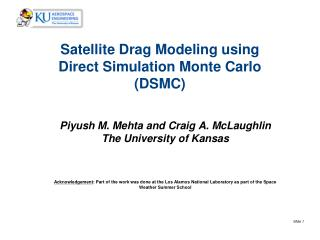# Satellite Drag Modeling using Direct Simulation Monte Carlo (DSMC) - PowerPoint PPT PresentationDownload PresentationSatellite Drag Modeling using Direct Simulation Monte Carlo (DSMC)

Presentation Description
Download Presentation## Satellite Drag Modeling using Direct Simulation Monte Carlo (DSMC)

- - - - - - - - - - - - - - - - - - - - - - - - - - - E N D - - - - - - - - - - - - - - - - - - - - - - - - - - -
##### Presentation Transcript

1. Satellite Drag Modeling using Direct Simulation Monte Carlo (DSMC) Piyush M. Mehta and Craig A. McLaughlin The University of Kansas Acknowledgement: Part of the work was done at the Los Alamos National Laboratory as part of the Space Weather Summer School

2. Introduction • Satellite Drag Model • Sources of error: Density, Drag Coefficient and Area • Density Modeling: • Typically uses constant drag coefficient to derive densities from satellite data • High Accuracy Satellite Drag Model (HASDM) uses drag coefficients varying with altitude • Drag Coefficient Modeling: • Orbit Prediction and Conjunction Analysis typically uses a constant drag coefficient

3. Density Mehta et al., 2011

4. Drag Coefficient • Drag Coefficient is a strong function of: • Energy Accommodation (model) • Gas Surface Interactions (GSI) (model) • Attitude • Surface Geometry • Atmospheric Composition and Temperature (NRLMSISE-00) • Surface Temperatures (Equations in Brown, AIAA Education Series, 2002) • Spacecraft Relative Velocity

5. Analytical Solution Sentman, 1961, Bird, 1994, Pilinski et al., 2011

6. DSMC DS3V

7. Energy Accommodation Defined as the fraction of the energy lost by free stream molecules on spacecraft surface impact Pilinski et al., 2010

8. Gas Surface Interaction (GSI) Sentman, 1961, Schamberg, 1959, Pilinski et al., 2011

9. Results Each data point is a DSMC simulation Each simulation take between 3-5 hrs depending on the machine Reference Simulation Conditions Tatm = 1157 K Tsc = 300 K Vr = 7590 m/s Molecular mass (m) = 11.35 amu

10. Results

11. Results

12. GRACE Altitude: 485 km at launch Eccentricity: <0.005 Inclination: 89 deg Mass: 432 kg

13. GRACE model for DSMC

14. Grace: All Models

15. Grace: Mesh for DS3V

16. GRACE DSMC Results July 19, 2005

17. GRACE DSMC Results compared with Sutton July 19, 2005

18. Drag Coefficient Modeling for GRACE Correlation Coefficients Data from July 19, 2005

19. Drag Coefficient Modeling for GRACE • Various curve fits were use for both Pressure and Shear drag contributions • Additional simulations performed at random times to validate models. • Error in using all the the models <1% • More simulations need to be performed at different space weather conditions for a complete model.

20. Conclusion: Drag Coefficient Modeling • The Direct Simulation Monte Carlo (DSMC) technique performed well in explicitly calculating drag coefficients for satellites with simple (sphere and cylinder) and complex geometries with complete and partial accommodation. • Results show strong correlation of the total drag coefficient for a sphere with energy-accommodation, spacecraft relative velocity, and free-stream atmospheric temperature. • Drag coefficients can vary by more than 20% for complex geometries and by as much as 10% for a sphere along the satellite orbit. Therefore, use of a constant drag coefficient should be avoided in deriving densities from orbit data or for satellite conjunction. • Drag coefficients calculated by Sutton lie within the extreme cases of attitude simulated for GRACE. A high fidelity drag coefficient model for GRACE is highly feasible.

21. Future Work • Create and validate GRACE Drag Coefficient model • Create Drag Coefficient Models for other satellites • Use the drag coefficient model to update density models • Work on ways to improve model fidelity

22. Questions?